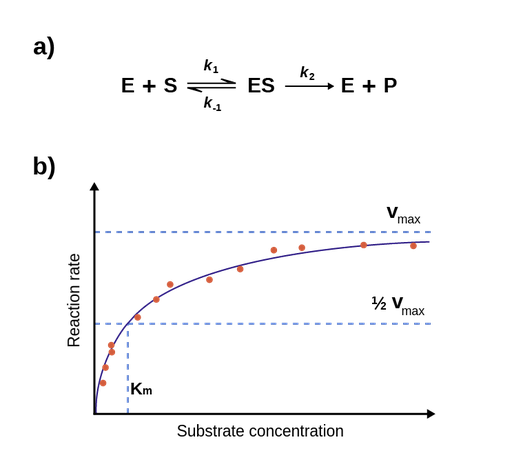The Michaelis-Menten model is a simple model of an enzymatic reaction developed by Leonor Michaelis and Maud Menten in 1913. The model is based on the following 2 assumptions:

• An enzymatic reaction proceeds in 2 steps: formation of an enzyme-substrate complex, ES, and dissociation of the enzyme and the product.
• After a (very) short period of time, the concentration of the ES complex reaches a steady state, where the rate of formation of ES equals the rate of its consumption.

The first assumption implies that the enzymatic reaction is made up of 4 different reactions: formation of ES from E and S, dissociation of ES into E and S, dissociation of ES into E and P, and formation of ES from E and P. The rate of a reaction is usually measured in the beginning of the reaction, where no significant amount of P has been formed; therefore, the rate of formation of ES from E and P can be ignored. This results in the following overall reaction (Figure 1.a) .Figure 1: Figure 1.a: Overall enzymatic reaction; Figure 1.b: Plot of initial rates of an enzymatic reaction plotted against the substrate concentration, and a Michaelis-Menten curve fitted to this plot. At low substrate concentrations, the curve is steep; however, at higher concentrations, the curve reaches a plateau, and the rate approaches Vmax. The interpretation of km is also clear from the figure; km is equal to the substrate concentration where the reaction rate is ½ • Vmax. 

This reaction implies that the rate of formation of products, i.e., the reaction rate, is given by V = k2 • [ES]. When almost all the enzyme is part of the enzyme-substrate complex, the reaction approaches its maximum velocity (Vmax). In the above reaction, k2 is the rate-limiting step, and Vmax can therefore be expressed as [E] • k2. The rate-limiting rate constant is also called kcat, or the turnover number, and in the above reaction, kcat = k2. This means, that Vmax = kcat • [E]. 

1. Lehninger, Albert L.; Nelson, David L.; Cox, Michael M. (2008). Principles of Biochemistry (5th ed.). New York, NY: W.H. Freeman and Company. ISBN 978-0-7167-7108-1.

Theory overview